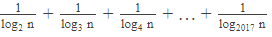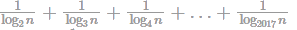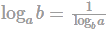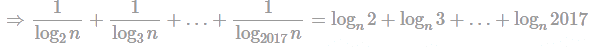If n = (2017)! then what is equal to?Corr...

### Related TestIf n = (2017)! then what isequal to?Kunaal SatijaGiven: n = (2017)!
To find:Using= logn(2017!)
= logn(n) = 1
Hence, the correct answer is 1.View courses related to this question Explore CAT courses
 Explore CAT coursesView courses related to this question1 Crore+ students have signed up on EduRev. Have you?

• ### Indias GDP per capita (in terms of purchasing power parity) almost doubled ... more(Scan QR code)